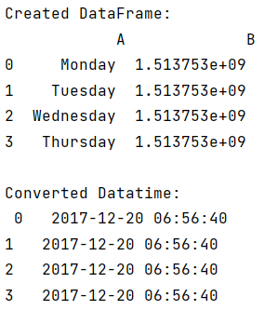# How to convert epoch time to datetime in pandas?

Given a Pandas DataFrame, we have to convert epoch time to datetime.
Submitted by Pranit Sharma, on June 15, 2022

Pandas is a special tool that allows us to perform complex manipulations of data effectively and efficiently. Inside pandas, we mostly deal with a dataset in the form of DataFrame. DataFrames are 2-dimensional data structures in pandas. DataFrames consist of rows, columns, and the data. The Data inside the DataFrame can be of any type.

## What is Epoch Time?

Epoch time or Unix time is the standard convention for representing real-world time. The epoch time or the real world time starts at 00:00:00 UTC where UTC stands for Coordinated Universal Time.

## Problem statement

Given a Pandas DataFrame whose column values are in epoch time format, we have to convert epoch time to datetime.

## Converting epoch time to datetime in pandas

To convert this epoch time into the readable date-time format, we will use pandas.to_datetime() and we will pass our required column along with another parameter unit = 's' inside this method.

Note

To work with pandas, we need to import pandas package first, below is the syntax:

```import pandas as pd
```

Let us understand with the help of an example,

## Python program to convert epoch time to datetime

```# Importing pandas package
import pandas as pd

# Creating a dictionary
d = {
'A':['Monday','Tuesday','Wednesday','Thursday'],
'B':[1.513753e+09,1.513753e+09,1.513753e+09,1.513753e+09]
}

# Creating a DataFrame
df = pd.DataFrame(d)

print("Created DataFrame:\n",df,"\n")

# Converting Date format
result = pd.to_datetime(df['B'],unit='s')

# Display result
print("Converted Datatime:\n",result)
```

## Output

The output of the above program is: